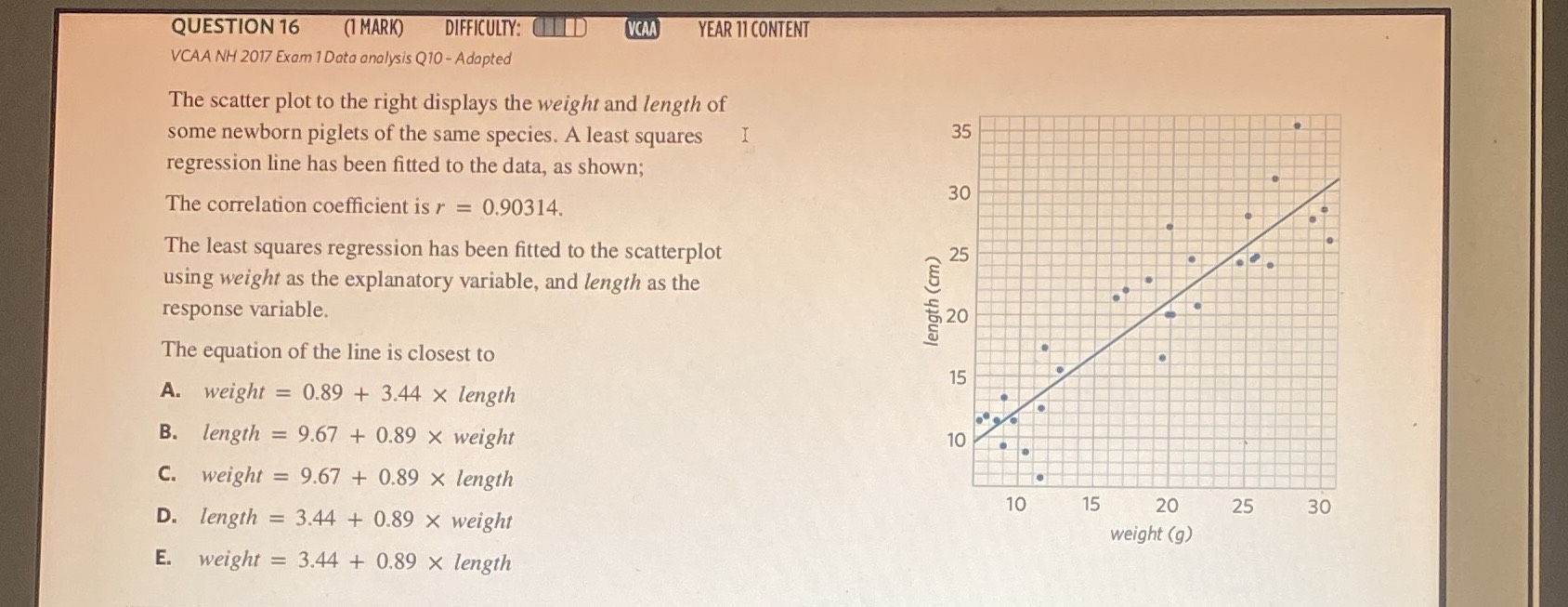### ¿Todavía tienes preguntas de matemáticas?

Pregunte a nuestros tutores expertos
Algebra
PreguntaThe scatter plot to the right displays the weight and length of some newborn piglets of the same species. A least squares regression line has been fitted to the data, as shown; The correlation coefficient is $$r = 0.90314 .$$

The least squares regression has been fitted to the scatterplot using weight as the explanatory variable, and length as the response variable. The equation of the line is closest to

A. weight $$= 0.89 + 3.44 \times$$ length

B. length $$= 9.67 + 0.89 \times$$ weight

C. weight $$= 9.67 + 0.89 \times$$ length

D. length $$= 3.44 + 0.89 \times$$ weight

E. weight $$= 3.44 + 0.89 \times$$ length